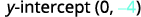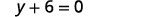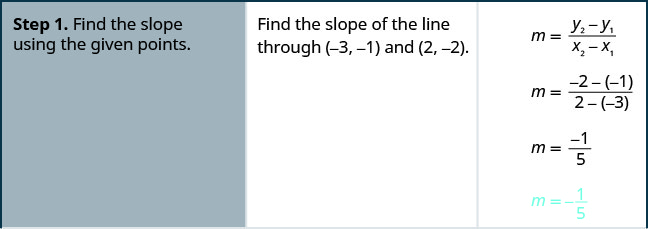# 3.4: 求直线方程

•• OpenStax
• OpenStax
$$\newcommand{\vecs}{\overset { \rightharpoonup} {\mathbf{#1}} }$$ $$\newcommand{\vecd}{\overset{-\!-\!\rightharpoonup}{\vphantom{a}\smash {#1}}}$$$$\newcommand{\id}{\mathrm{id}}$$ $$\newcommand{\Span}{\mathrm{span}}$$ $$\newcommand{\kernel}{\mathrm{null}\,}$$ $$\newcommand{\range}{\mathrm{range}\,}$$ $$\newcommand{\RealPart}{\mathrm{Re}}$$ $$\newcommand{\ImaginaryPart}{\mathrm{Im}}$$ $$\newcommand{\Argument}{\mathrm{Arg}}$$ $$\newcommand{\norm}{\| #1 \|}$$ $$\newcommand{\inner}{\langle #1, #2 \rangle}$$ $$\newcommand{\Span}{\mathrm{span}}$$ $$\newcommand{\id}{\mathrm{id}}$$ $$\newcommand{\Span}{\mathrm{span}}$$ $$\newcommand{\kernel}{\mathrm{null}\,}$$ $$\newcommand{\range}{\mathrm{range}\,}$$ $$\newcommand{\RealPart}{\mathrm{Re}}$$ $$\newcommand{\ImaginaryPart}{\mathrm{Im}}$$ $$\newcommand{\Argument}{\mathrm{Arg}}$$ $$\newcommand{\norm}{\| #1 \|}$$ $$\newcommand{\inner}{\langle #1, #2 \rangle}$$ $$\newcommand{\Span}{\mathrm{span}}$$$$\newcommand{\AA}{\unicode[.8,0]{x212B}}$$

##### 学习目标

• 给定斜率和 y 截距，求直线方程
• 在给定斜率和点的情况下找出直线的方程
• 在给定两点的情况下找出直线的方程
• 求一条平行于给定直线的直线的方程
• 求一条垂直于给定直线的直线的方程

1. 解决：$$\frac{2}{5}(x+15)$$
如果你错过了这个问题，请查看 [链接]
2. 简化：$$−3(x−(−2))$$
如果你错过了这个问题，请查看 [链接]
3. 求解 y:$$y−3=−2(x+1)$$
如果你错过了这个问题，请查看 [链接]

## 给定斜率和 y 截距求直线方程

##### 示例$$\PageIndex{1}$$

 命名斜坡。命名为 y 截距。将这些值替换为$$y=mx+b$$。##### 示例$$\PageIndex{2}$$

$$y=\frac{2}{5}x+4$$

##### 示例$$\PageIndex{3}$$

$$y=−x−3$$

##### 示例$$\PageIndex{4}$$y 截距为$$(0,−4)$$，图形通过$$(3,−2)$$

 通过计算上升和跑步来找到斜率。找出 y 截距。将这些值替换为 y=mx+b.y=mx+b。##### 示例$$\PageIndex{5}$$$$y=\frac{3}{5}x+1$$

##### 示例$$\PageIndex{6}$$$$y=\frac{4}{3}x−5$$

## 在给定斜率和点的情况下求直线方程

$$\begin{array} {llll} {} &{m} &= &{\frac{y-y_1}{x-x_1}} \\ {\text{Multiply both sides of the equation by }x−x_1.} &{m(x-x_1)} &= &{\left( \frac{y−y_1}{x−x_1} \right)(x−x_1)} \\ {\text{Simplify.}} &{m(x-x_1)} &= &{y-y_1} \\ {\text{Rewrite the equation with theyterms on the left.}} &{y-y_1} &= &{m(x-x_1)} \\ \end{array}$$

##### 直线方程的点斜率形式

$y−y_1=m(x−x_1) \nonumber$

##### 示例$$\PageIndex{7}$$##### 示例$$\PageIndex{8}$$

$$y=−\frac{2}{5}x−1$$

##### 示例$$\PageIndex{9}$$

$$y=−\frac{3}{4}x−4$$

##### 在给定斜率和点的情况下找出直线的方程。
1. 确定斜率。
2. 找出重点。
3. 将这些值替换为点斜率形式，即$$y−y_1=m(x−x_1)$$
4. 以斜率截距形式写出方程。
##### 示例$$\PageIndex{10}$$

 确定斜率。找出重点。将这些值替换为 y−y1=m (x−x1) .y−y1=m (x−x1)。简化。以斜率截距形式书写。 它采用 y 格式，但可以写入$$y=0x−6$$。

##### 示例$$\PageIndex{11}$$

$$y=8$$

##### 示例$$\PageIndex{12}$$

$$y=4$$

## 给定两点求直线方程

##### 示例$$\PageIndex{13}$$##### 示例$$\PageIndex{14}$$

$$y=\frac{1}{3}x−\frac{10{3}$$

##### 示例$$\PageIndex{15}$$

$$y=−\frac{2}{5}x−\frac{23}{5}$$

##### 求给定两个点的直线的方程。
1. 使用给定的点找到斜率。 $$m=\frac{y_2−y_1}{x_2−x_1}$$
2. 选择一个点。
3. 将这些值替换为点斜率形式：$$y−y_1=m(x−x_1)$$
4. 以斜率截距形式写出方程。
##### 示例$$\PageIndex{16}$$

$m=\frac{y_2−y_1}{x_2−x_1} \nonumber$

$m=\frac{4−5}{−3−(−3)} \nonumber$

$m=\frac{−1}{0} \nonumber$

##### 示例$$\PageIndex{17}$$

$$x=5$$

##### 示例$$\PageIndex{18}$$

$$x=−4$$

## 求一条平行于给定直线的直线的方程##### 示例$$\PageIndex{19}$$##### 示例$$\PageIndex{20}$$

$$y=3x−10$$

##### 示例$$\PageIndex{21}$$

$$y=\frac{1}{2}x+1$$

##### 求一条平行于给定直线的直线的方程。
1. 找出给定直线的斜率。
2. 找出平行线的斜率。
3. 找出重点。
4. 将这些值替换为点斜率形式：$$y−y_1=m(x−x_1)$$
5. 以斜率截距形式写出方程。

## 求一条垂直于给定直线的直线的方程$y=2x−3 perpendicular line \nonumber$

$m=2 m⊥=−12\nonumber$##### 示例$$\PageIndex{22}$$##### 示例$$\PageIndex{23}$$

$$y=−\frac{1}{3}x+\frac{10}{3}$$

##### 示例$$\PageIndex{24}$$

$$y=−2x+16$$

##### 求一条垂直于给定直线的直线的方程。
1. 找出给定直线的斜率。
2. 找出垂直线的斜率。
3. 找出重点。
4. 将这些值替换为点斜率形式，即$$y−y_1=m(x−x_1)$$
5. 以斜率截距形式写出方程。

##### 示例$$\PageIndex{25}$$

$$y=−5$$

##### 示例$$\PageIndex{26}$$

$$y=−1$$##### 示例$$\PageIndex{28}$$

$$x=−5$$

##### 示例$$\PageIndex{29}$$

$$x=−4$$

## 关键概念

• 如何在给定斜率和点的情况下找到直线方程。
1. 确定斜率。
2. 找出重点。
3. 将这些值替换为点斜率形式，即$$y−y_1=m(x−x_1)$$
4. 以斜率截距形式写出方程。

• 如何在给定两个点的情况下找到一条直线的方程。
1. 使用给定的点找到斜率。 $$m=\frac{y_2−y_1}{x_2−x_1}$$
2. 选择一个点。
3. 将这些值替换为点斜率形式：$$y−y_1=m(x−x_1)$$
4. 以斜率截距形式写出方程。
写出直线方程
如果给出： 使用： 表单：
斜率和 y 截距 斜率截距 $$y=mx+b$$
斜率和点 点斜率 $$y−y_1=m(x−x_1)$$
两点积分 点斜率 $$y−y_1=m(x−x_1)$$
• 如何找到平行于给定直线的直线的方程。
1. 找出给定直线的斜率。
2. 找出平行线的斜率。
3. 找出重点。
4. 将这些值替换为点斜率形式：$$y−y_1=m(x−x_1)$$
5. 以斜率截距形式写出方程
• 如何找到垂直于给定直线的直线的方程。
1. 找出给定直线的斜率。
2. 找出垂直线的斜率。
3. 找出重点。
4. 将这些值替换为点斜率形式，即$$y−y_1=m(x−x_1)$$
5. 以斜率截距形式写出方程。